# Types of Chemical Reactions Predicting Products from the

• Slides: 19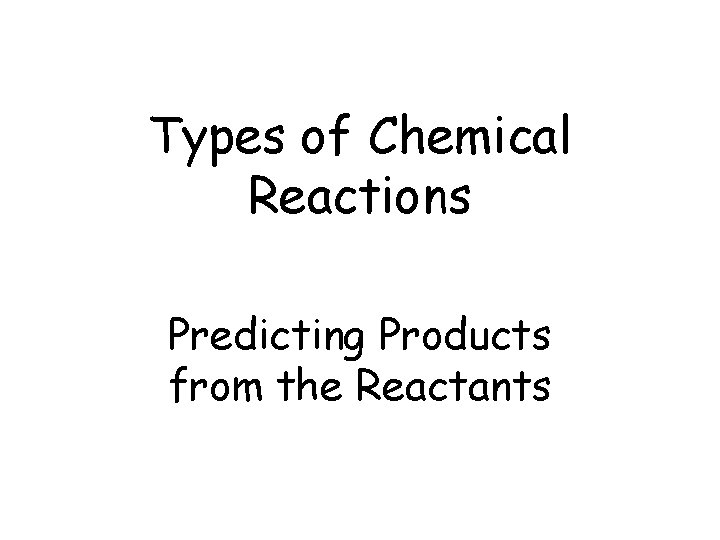Types of Chemical Reactions Predicting Products from the Reactants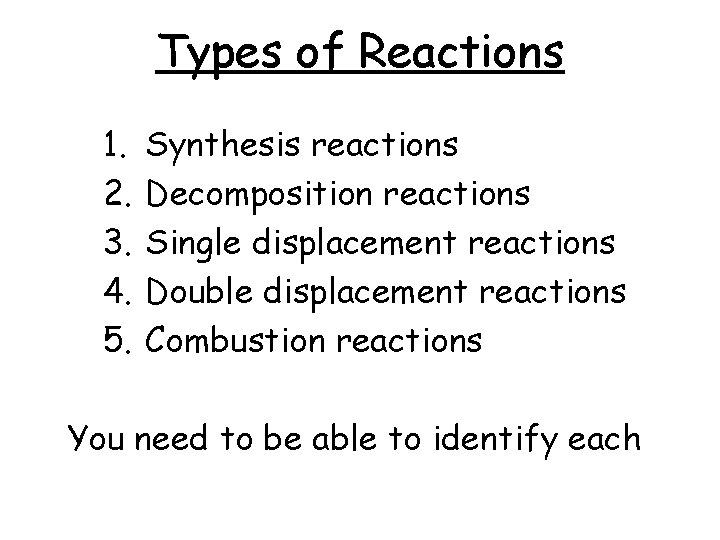Types of Reactions 1. 2. 3. 4. 5. Synthesis reactions Decomposition reactions Single displacement reactions Double displacement reactions Combustion reactions You need to be able to identify each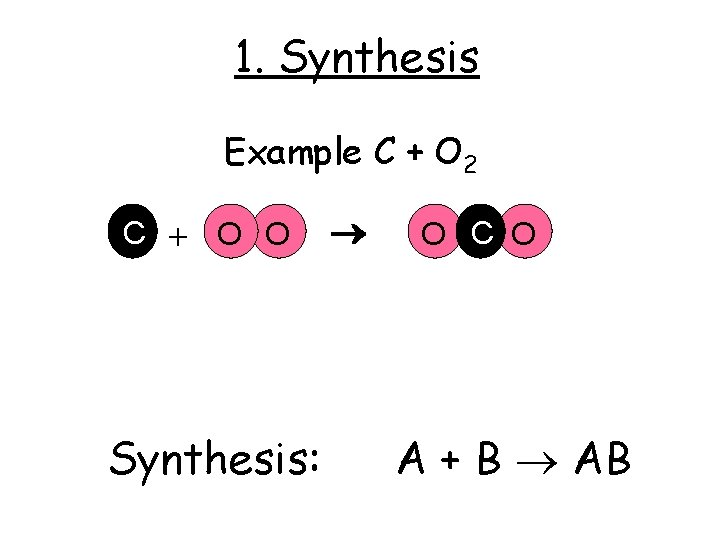1. Synthesis Example C + O 2 C + O O Synthesis: O C O A + B ABCriss Cross the Charges to Balance • Mg + N ? • Mg = +2 • N = -3 +2 • Mg + -3 N Mg 3 N 2 • Synthesis: A + B = ABEx. Synthesis ReactionPractice • Predict the products: 2 Na(s) + Cl 2(g) 2 Na. Cl(s) Mg(s) + F 2(g) Mg. F 2(s) 2 Al(s) + 3 F 2(g) 2 Al. F 3(s) • Now, balance them. (Criss-Cross!)But WAIT! What Does the (s) and (aq) and (g) Mean? ? ? • (s) in a chemical equation signifies a SOLID • (g) in a chemical equation is a GAS • (aq) means “aqueous” (LIQUID) • Why do you need to note that? Because sometimes …a phase can change in a reaction!2. Decomposition Example: Na. Cl Cl Na General: Cl + Na AB A + B Compound = Element + Element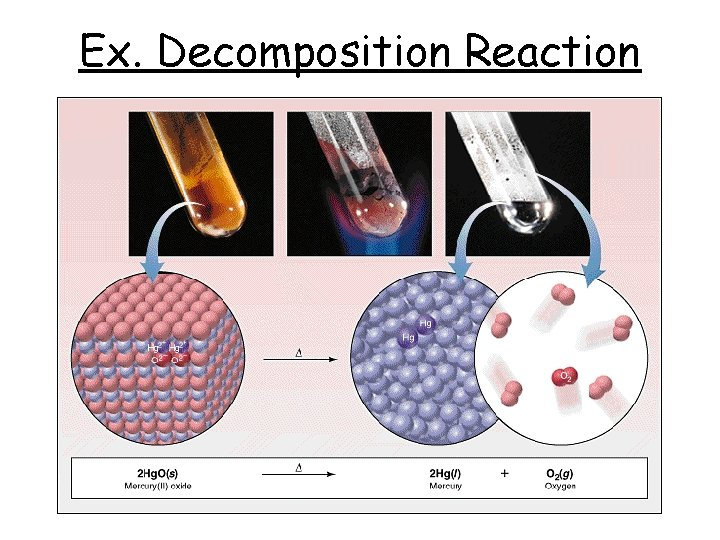Ex. Decomposition Reaction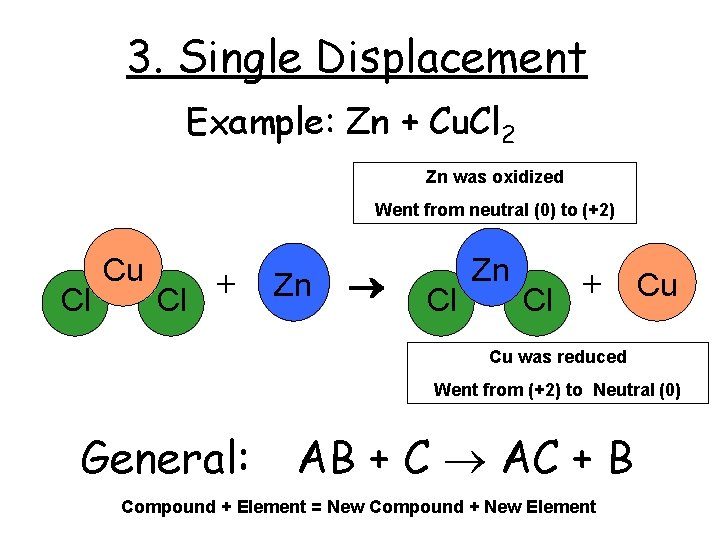3. Single Displacement Example: Zn + Cu. Cl 2 Zn was oxidized Went from neutral (0) to (+2) Cl Cu + Cl Zn + Cu Cl Cu was reduced Went from (+2) to Neutral (0) General: AB + C AC + B Compound + Element = New Compound + New Element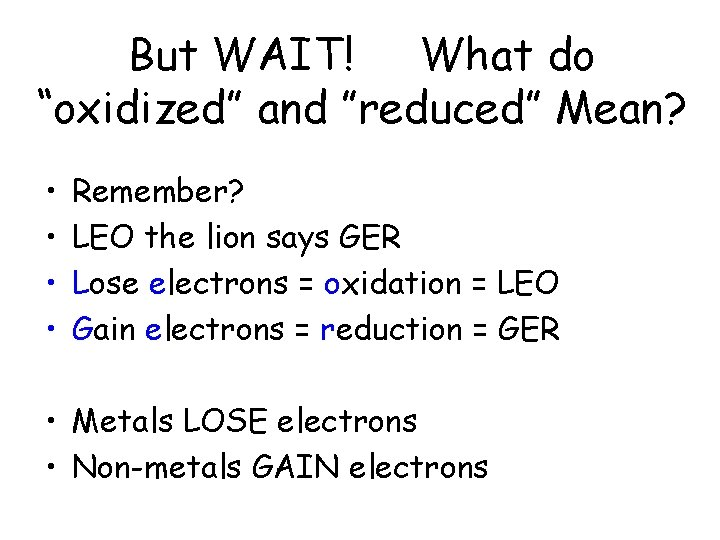But WAIT! What do “oxidized” and ”reduced” Mean? • • Remember? LEO the lion says GER Lose electrons = oxidation = LEO Gain electrons = reduction = GER • Metals LOSE electrons • Non-metals GAIN electronsEx. Single Replacement ReactionSingle Replacement Reactions • Write and balance the following single replacement reaction equations: • Zn(s) + 2 HCl(aq) Zn. Cl 2 + H 2(g) • 2 Na. Cl(s) + F 2(g) 2 Na. F(s) + Cl 2(g) • 2 Al(s)+ 3 Cu(NO 3)2(aq) 3 Cu(s)+ 2 Al(NO 3)3(aq)4. Double Displacement Example: Mg. O + Ca. S Mg O + Ca General: S Mg S + Ca O AB + CD AD + CB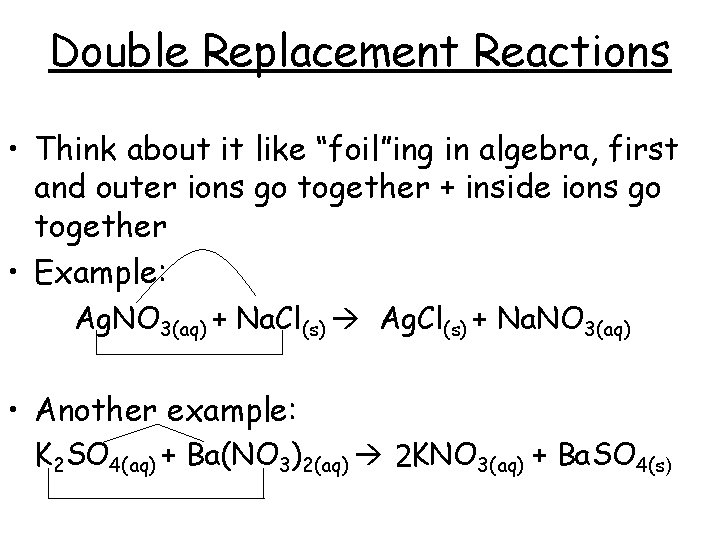Double Replacement Reactions • Think about it like “foil”ing in algebra, first and outer ions go together + inside ions go together • Example: Ag. NO 3(aq) + Na. Cl(s) Ag. Cl(s) + Na. NO 3(aq) • Another example: K 2 SO 4(aq) + Ba(NO 3)2(aq) 2 KNO 3(aq) + Ba. SO 4(s)Practice • Predict the products: 1. 2. 3. 4. 5. HCl(aq) + Ag. NO 3(aq) Ca. Cl 2(aq) + Na 3 PO 4(aq) Pb(NO 3)2(aq) + Ba. Cl 2(aq) Fe. Cl 3(aq) + Na. OH(aq) H 2 SO 4(aq) + Na. OH(aq) 6. KOH(aq) + Cu. SO 4(aq)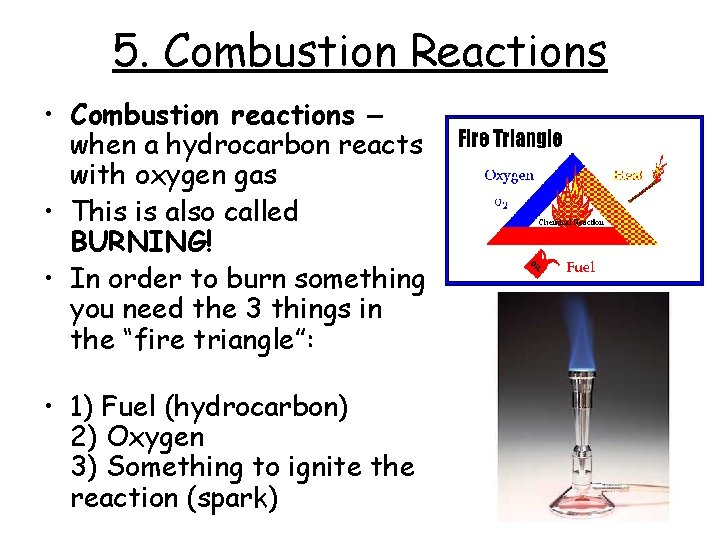5. Combustion Reactions • Combustion reactions – when a hydrocarbon reacts with oxygen gas • This is also called BURNING! • In order to burn something you need the 3 things in the “fire triangle”: • 1) Fuel (hydrocarbon) 2) Oxygen 3) Something to ignite the reaction (spark)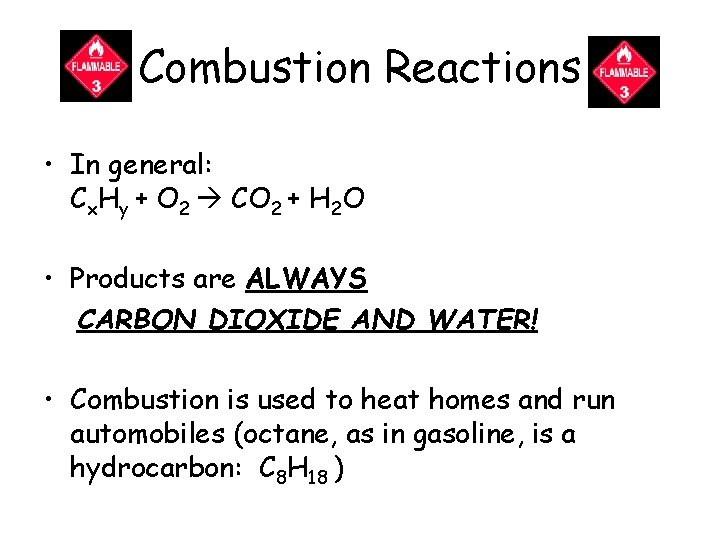Combustion Reactions • In general: Cx. Hy + O 2 CO 2 + H 2 O • Products are ALWAYS CARBON DIOXIDE AND WATER! • Combustion is used to heat homes and run automobiles (octane, as in gasoline, is a hydrocarbon: C 8 H 18 )Mixed Practice • State the type of reaction & predict the products (try to balance the equation!) 1. 2. 3. 4. 5. Ba. Cl 2 + H 2 SO 4 C 6 H 12 + O 2 Zn + Cu. SO 4 Cs + Br 2 Fe. CO 3